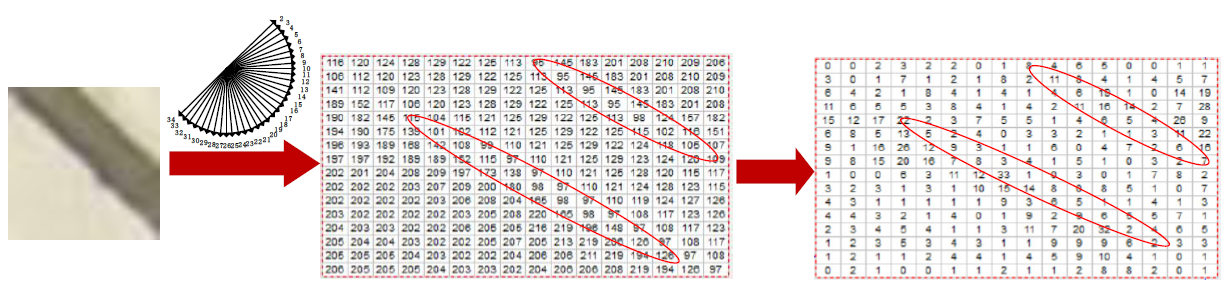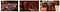# Review — DLT: Deep Learning-Based Nonlinear Transform for HEVC Intra Coding (HEVC Intra)

## Deep Learning-based Transform (DLT) Using Autoencoder, 0.75% BD-Rate Reduction is Achieved

• The intra prediction signal is utilized as side information to reduce the directionality in the residual.
• A novel loss function is used to characterize the efficiency of the transform during the training.

# Outline

1. Directionality in Residual Domain
2. DLT: Network Architecture
3. Loss Function
4. Experimental Results

# 1. Directionality in Residual DomainThe directional information in the prediction block and the residual block after directional intra prediction.
• After getting the difference between the original block and the prediction block, as shown above (right), DCT transformed and quantized, there is still directional information in the residual domain.
• That means DCT cannot decorrelate the block well to compact the energy.
• Thus, CNN is designed to solve this problem, as shown in the first figure at the top of this story.
• It is noted that Deep Learning-based Transform (DLT) is only applied onto 8×8 blocks.
• The flag DLT_Flag is transmitted to the decoder for each 8×8 transform unit (TU) to inform whether the DLT is used or not.

# 2. DLT: Network Architecture

## 2.1. Directional Module (Top)

• All of these layers use the tanh activation function.
• Before feeding to the neural network, the mean is substracted from the prediction.
• Finally, the directional information Idir, is extracted.

## 2.2. Transform Module (Bottom)

• Autoencoder architecture is used as the transform.
• The encoder and decoder are both composed of 1 convolutional layer and initialized using the DCT basis functions.

## 2.2.1. Encoder for Forward Transform

• The encoder of the Autoencoder is adopted to perform the forward transform.
• To eliminate the directionality in the residuals, the difference between the residual Xres and extracted directional information Idir is used as the input of the encoder:
• The number of the transform coefficients equals the size of input Xres.

## 2.2.2. Decoder for Inverse Transform

• For the inverse transform, the decoder of the Autoencoder takes the transform coefficients Z as the input.
• To recover the directionality of the residual, the extracted directional information Idir is added back to output of the decoder.
• Furthermore, to compact the signal energy into a few coefficients, only K transform coefficients in Z are used to perform the inverse transform. In the implementation, K = 8:

# 3. Loss Function

## 3.1. L2 Loss

• A pixel-wise L2 loss is used:

## 3.2. Energy Compact Loss

• The transform coding gain is widely adopted to measure the amount of energy compaction achieved by the transform.
• It is defined as a ratio of the arithmetic mean to the geometric mean of the variances of the transform coefficients:

## 3.3. Total Loss

• The total loss L is the weighted sum of L2 loss and energy compact loss:

# 4. Experimental Results

## 4.1. Training

• Uncompressed Color Image Database (UCID) is used as training set.
• HEVC (HM-16.20) is used to compress the images in the UCID under default configuration, and mark all the 4×4 TUs.
• Then, the minimum transform size is set to 8 and the images are compressed again.
• At the same time, the 8 × 8 TUs where all its 4 × 4 TUs are marked at the first step are extracted. In this way, the complex 8×8 TUs where the DCT has a poor performance, can be extracted.
• Only luma is considered, and all the values are normalized to the range [-1, 1] by dividing by 255.
• Only one model is trained for four QPs {22, 27, 32, 37}.

## 4.2. BD-Rate

• Higher performance can be achieved for these test sequences with abundant textures, e.g., ParkScene, RaceHorses.

## 4.3. UsageThe red blocks represent the 8×8 TUs that use the proposed DLT instead of the DCT
• It illustrates that the proposed DLT can capture the non-stationary at the textural area of the natural video.

PhD, Researcher. I share what I've learnt and done. :) My LinkedIn: https://www.linkedin.com/in/sh-tsang/, My Paper Reading List: https://bit.ly/33TDhxG

## More from Sik-Ho Tsang

PhD, Researcher. I share what I've learnt and done. :) My LinkedIn: https://www.linkedin.com/in/sh-tsang/, My Paper Reading List: https://bit.ly/33TDhxG